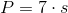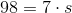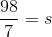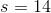# ISEE Upper Level Math : How to find the length of a side in other polygons

## Example Questions

### Example Question #1 : How To Find The Length Of A Side In Other Polygons

The perimeter of a regular octagon is one mile. Give the sidelength in feet.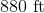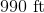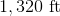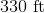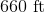Explanation:

One mile is equal to 5,280 feet, so divide this by 8: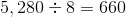feet

### Example Question #30 : Geometry

What is the sidelength, in feet and inches, of a regular octagon with perimeter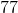feet?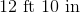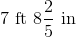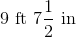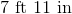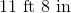Explanation:feet is equivalent to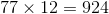inches. Each side of a regular octagon, an eight-sided figure, measures one-eighth of this:

Each side of an octagon with perimeterfeet measures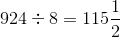inches.

Since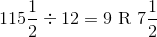,

this is equal to.

### Example Question #31 : Geometry

In a septagon, each of thesides are equal to one another. If the perimeter is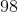feet, what is the length of one of the sides?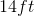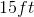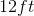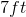Explanation:

If the perimeter of a septagon, in which each side is equal to the other, is 98, the length of each side will be 7 feet because 98 divided by 7 is 14.